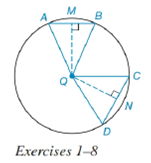Chapter 6.4, Problem 1EElementary Geometry For College St...

7th Edition
Alexander + 2 others
ISBN: 9781337614085

Solutions

Chapter
SectionElementary Geometry For College St...

7th Edition
Alexander + 2 others
ISBN: 9781337614085
Textbook Problem

In Exercises 1 to 8, use the figure provided.If m C D ⌢ < m A B ⌢ , write an inequality that compares m ∠ C Q D and m ∠ A Q B .To determine

To write:

An inequality that compares mCQD and mAQB.

Explanation

Given:

mCD<mAB and the figure given below

Theorem:

In a circle (or in congruent circles) containing two unequal arcs, the larger arc corresponds to the larger central angle.

Calculation:

Using the theorem.

Since the arcs are unequal i.e. mCD<mAB and from the given figure

Still sussing out bartleby?

Check out a sample textbook solution.

See a sample solution

The Solution to Your Study Problems

Bartleby provides explanations to thousands of textbook problems written by our experts, many with advanced degrees!

Get Started

Fill in each blank: 36mi=yd

Elementary Technical Mathematics

In Exercise 25-30, find the rules for the composite functions f g and g f. 27. f(x) = x+1; g(x) = x2 1

Applied Calculus for the Managerial, Life, and Social Sciences: A Brief Approach

Simplify the expressions in Exercises 97106. 41/147/2

Finite Mathematics and Applied Calculus (MindTap Course List)

Test the series for convergence or divergence. 9. n=1(1)nen

Single Variable Calculus: Early Transcendentals

True or False: The graph of x = 5 is a cylinder.

Study Guide for Stewart's Multivariable Calculus, 8th